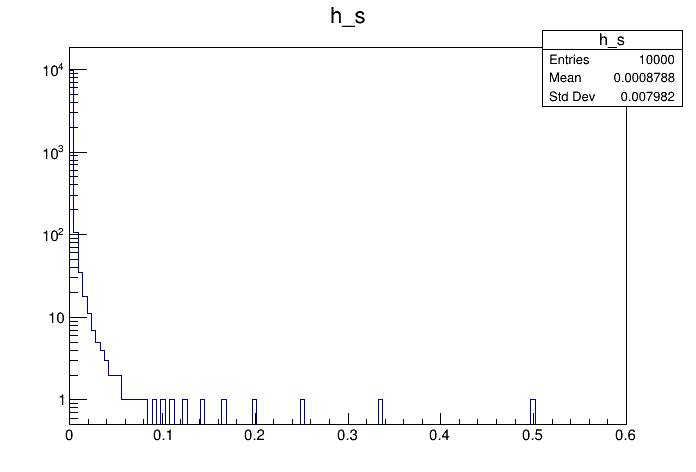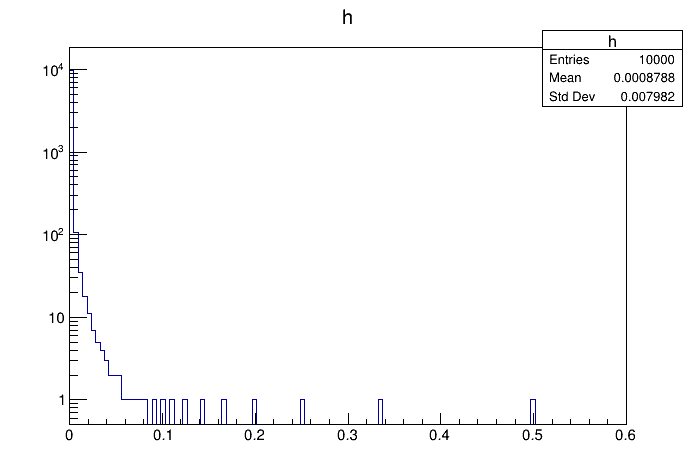# Df 0 1 1_ R O O T Data Source¶

This tutorial illustrates how use the RDataFrame in combination with a RDataSource. In this case we use a RRootDS. This data source allows to read a ROOT dataset from a RDataFrame in a different way, not based on the regular RDataFrame code. This allows to perform all sorts of consistency checks and illustrate the usage of the RDataSource in a didactic context.

Author: Danilo Piparo
This notebook tutorial was automatically generated with ROOTBOOK-izer from the macro found in the ROOT repository on Monday, January 27, 2020 at 01:07 AM.

In :
using TDS = ROOT::RDF::RDataSource;


A helper function is created:

In :
%%cpp -d
void fill_tree(const char *treeName, const char *fileName)
{
ROOT::RDataFrame d(10000);
auto i = 0.;
d.Define("b1", [&i]() { return i++; }).Snapshot(treeName, fileName);
}

In :
auto fileName = "df011_ROOTDataSources.root";
auto treeName = "myTree";
fill_tree(treeName, fileName);

auto d_s = ROOT::RDF::MakeRootDataFrame(treeName, fileName);


/ now we have a regular rdataframe: the ingestion of data is delegated to / the RDataSource. At this point everything works as before.

In :
auto h_s = d_s.Define("x", "1./(b1 + 1.)").Histo1D({"h_s", "h_s", 128, 0, .6}, "x");


/ now we redo the same with a rdf and we draw the two histograms

In :
ROOT::RDataFrame d(treeName, fileName);
auto h = d.Define("x", "1./(b1 + 1.)").Histo1D({"h", "h", 128, 0, .6}, "x");

auto c_s = new TCanvas();
c_s->SetLogy();
h_s->DrawClone();

auto c = new TCanvas();
c->SetLogy();
h->DrawClone();

return 0;


Draw all canvases

In :
gROOT->GetListOfCanvases()->Draw()